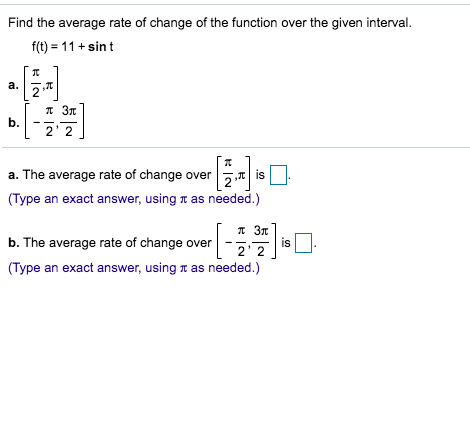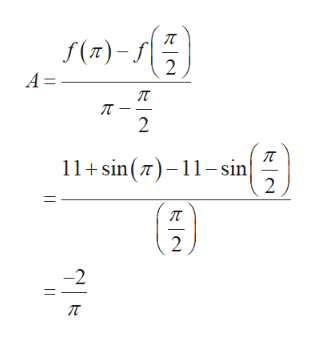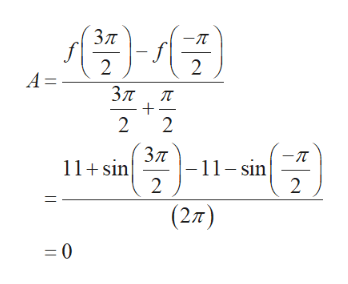Find the average rate of change of the function over the given intervalf(t) 11+sin ta.2п Зт3b.2 2a. The average rate of change overis(Type an exact answer, using T as needed.)3Tisb. The average rate of change over2(Type an exact answer, using T as needed.)

Question

Can I get help with this question step by step?help_outlineImage TranscriptioncloseFind the average rate of change of the function over the given interval f(t) 11+sin t a. 2 п Зт 3 b. 2 2 a. The average rate of change over is (Type an exact answer, using T as needed.) 3T is b. The average rate of change over 2 (Type an exact answer, using T as needed.) fullscreen
Step 1

Calculation:

a)

Compute the average rate of change of f(x) over [π/2, π] as follows.help_outlineImage Transcriptionclosef(T) - 2 A = 2 11+sin()sin| 2 2 -2 fullscreen
Step 2

b)

Compute the average rate of change of f(x) over [&mi...help_outlineImage TranscriptioncloseЗл 2 2 A = Зл 2 2 11sin 11-sin 2 2 (27) 0 fullscreen

Want to see the full answer?

See Solution

Want to see this answer and more?

Our solutions are written by experts, many with advanced degrees, and available 24/7

See Solution
Tagged in

Calculus